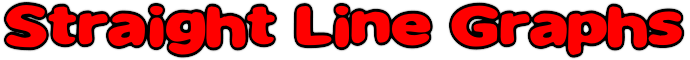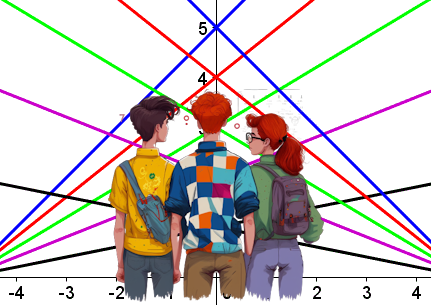This is a visual aid to help pupils learn about straight line graphs using a computer graph plotting program such as Autograph, an online Graph Plotter or a Graphic Display Calculator (GDC). The challenges are presented one at a time in increasing order of difficulty. Pupils need to reproduce the images projected on to the whiteboard using their graph plotting software.

If you are looking for the online, self-checking exercise on Straight Line Graphs it is here.

#### Straight Line Graph EquationAn online exercise about the equation y=mx+c and the features of a straight line graph.

Transum.org/go/?to=equationline

#### Graphs of Vertical and Horizontal Lines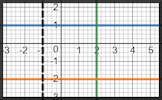Questions about the equations of straight line graphs that are parallel to the axes.

Transum.org/go/?to=vandh

#### Graph Patterns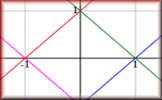Find the equations which will produce the given patterns of graphs.

 There is a printable worksheet to go with this activity.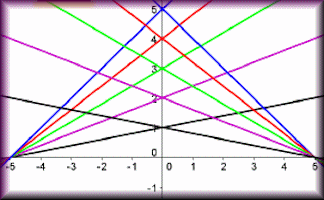An activity called Graph Patterns is perfect for pupils online.